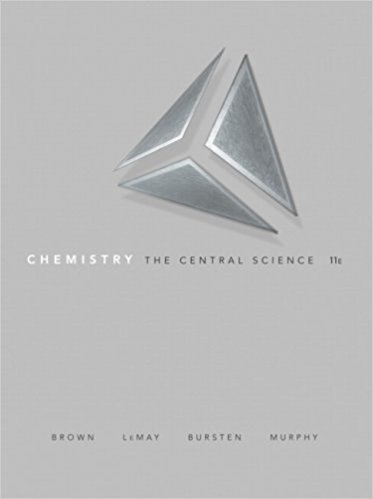×
×

# (a) If the core electrons were totally effective at shielding the valence electrons andISBN: 9780136006176 365

## Solution for problem 7.85 Chapter 7

Chemistry: The Central Science | 11th Edition

• Textbook Solutions
• 2901 Step-by-step solutions solved by professors and subject experts
• Get 24/7 help from StudySoup virtual teaching assistantsChemistry: The Central Science | 11th Edition

4 5 1 284 Reviews
19
5
Problem 7.85

(a) If the core electrons were totally effective at shielding the valence electrons and the valence electrons provided no shielding for each other, what would be the effective nuclear charge acting on the 3s and 3p valence electrons in P? (b) Repeat these calculations using Slater's rules. (c) Detailed calculations indicate that the effective nuclear charge is 5.6+ for the 3s electrons and 4.9+ for the 3p electrons. Why are the values for the 3s and 3p electrons different? (d) If you remove a single electron from a P atom, which orbital will it come from? Explain.

Step-by-Step Solution:
Step 1 of 3

CEM 141 Lecture 27: Introduction to Lewis Structures Dr. Jian Hu th November 7 , 2016 Review of Covalent Bonding  Valence electrons of one atom are constantly attracted to the nucleus of another  The nuclei of both atoms attract electrons as they are held in between the two nuclei, causing the two atoms to stay together as a unit  The electrostatic force that...

Step 2 of 3

Step 3 of 3

##### ISBN: 9780136006176

Since the solution to 7.85 from 7 chapter was answered, more than 217 students have viewed the full step-by-step answer. The answer to “(a) If the core electrons were totally effective at shielding the valence electrons and the valence electrons provided no shielding for each other, what would be the effective nuclear charge acting on the 3s and 3p valence electrons in P? (b) Repeat these calculations using Slater's rules. (c) Detailed calculations indicate that the effective nuclear charge is 5.6+ for the 3s electrons and 4.9+ for the 3p electrons. Why are the values for the 3s and 3p electrons different? (d) If you remove a single electron from a P atom, which orbital will it come from? Explain.” is broken down into a number of easy to follow steps, and 97 words. This textbook survival guide was created for the textbook: Chemistry: The Central Science , edition: 11. Chemistry: The Central Science was written by and is associated to the ISBN: 9780136006176. The full step-by-step solution to problem: 7.85 from chapter: 7 was answered by , our top Chemistry solution expert on 03/09/18, 07:03PM. This full solution covers the following key subjects: . This expansive textbook survival guide covers 25 chapters, and 2360 solutions.

Unlock Textbook Solution